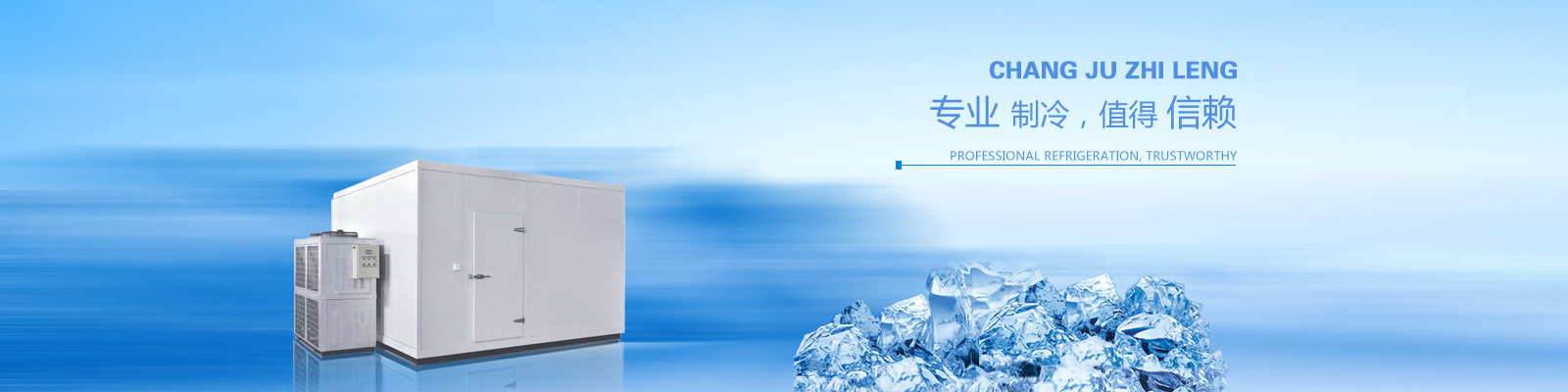# 欢迎浏览铜陵光大制冷设备有限公司网站，安徽冷库制冷设备，今天是： var enable = 0; today = new Date(); var day; var date; var time_start = new Date(); var clock_start = time_start.getTime(); if (today.getDay() == 0) day = "星期日" if (today.getDay() == 1) day = "星期一" if (today.getDay() == 2) day = "星期二" if (today.getDay() == 3) day = "星期三" if (today.getDay() == 4) day = "星期四" if (today.getDay() == 5) day = "星期五" if (today.getDay() == 6) day = "星期六" yr = today.getYear(); if (yr < 1000) yr += 1900; date = yr + "年" + (today.getMonth() + 1) + "月" + today.getDate() + "日 "; document.write("<span style='font-size: 9pt;color:#fff;'>" + date); document.write(day + "</font></span>");

### 联系我们| 在线留言••• 冷库制冷设备：保鲜库

• 冷库制冷设备：保鲜库

• 冷库制冷设备：铅管冷冻库

• 冷库制冷设备：机器设备

• 冷库制冷设备：压缩机

• 冷库制冷设备：压缩机

• 冷库制冷设备：压缩机

• 冷库制冷设备：压缩机

• 冷库制冷设备：压缩机

• 冷库制冷设备：压缩机

• 冷库制冷设备：压缩机

• 冷库制冷设备：压缩机